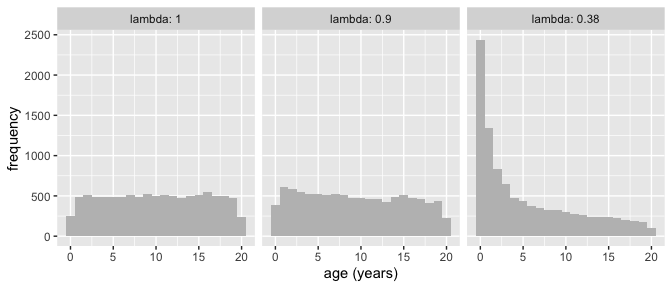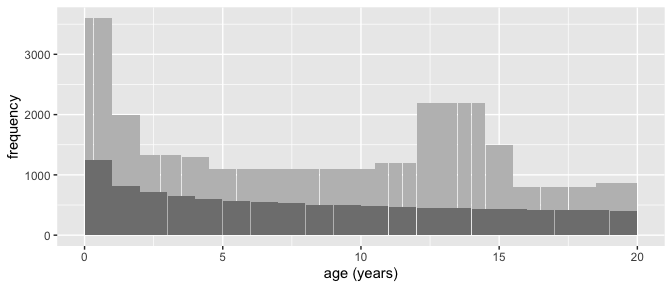# Sample size and sample composition

This vignette describes two functions for estimating optimal sample size and sample composition in growth reference centile studies, based on the paper by Cole (2020).

There are two criteria that determine the optimal sample size N and sample composition $$\lambda$$ in centile studies:

• the centile of interest, which is expressed as a z-score z
• the required level of precision for that centile, expressed as the z-score standard error SEz

Take for example the Cuban Growth Study (Healy 1974, Jordan 1975), which was designed to estimate the 3rd height centile for boys aged 8 with a standard error (SE) of 0.3 cm.

The 3rd centile corresponds to z = -1.88, and the required SE in z-score units is obtained by dividing the SE by the height SD at age 8 of 5.75 cm. This gives the precision in z-score units as SEz = 0.3/5.75 = 0.053.

Cole (2020) pointed out that when calculating sample size it is essential to think also about sample composition, i.e. the age distribution of the measurements. All centile studies need to collect extra data in infancy compared to later in childhood, because babies grow faster than children. But just how much more depends on which centile is the focus. The median requires heavy infant oversampling, whereas the 0.4th centile where z = -2.67 needs very little.

The degree of oversampling is defined by the quantity $$\lambda$$, see Cole (2020), where $$\lambda$$ = 1 means uniform sampling, while for example $$\lambda$$ = 0.4 indicates heavy infant oversampling.

To run the examples, first load the tidyverse and sitar libraries.

## optimal_design

The optimal_design function takes as arguments z, lambda, N, SEz and age. It then calculates the optimal sample composition, based either on z or lambda, and the optimal sample size based either on N or SEz. If both N and SEz are given, N takes precedence. If both z and lambda are given, SEz also depends on age.

The return value is p, the centile corresponding to z, with its 95% confidence interval.

### Example

The following code estimates optimal lambda and SEz for nine centiles spaced two-thirds of a z-score apart, based on a sample of 10,000 children.

knitr::kable(optimal_design(z = -4:4*2/3, N = 10000), digits = c(2, 2, 0, 3, 0, 2, 2))
z lambda N SEz age p plo phi
-2.67 0.90 10000 0.065 10 0.4th 0.26 0.56
-2.00 0.76 10000 0.054 10 2nd 1.75 2.93
-1.33 0.60 10000 0.044 10 9th 7.75 10.66
-0.67 0.45 10000 0.037 10 25th 22.97 27.64
0.00 0.38 10000 0.033 10 50th 47.34 52.66
0.67 0.45 10000 0.037 10 75th 72.36 77.03
1.33 0.60 10000 0.044 10 91st 89.34 92.25
2.00 0.76 10000 0.054 10 98th 97.07 98.25
2.67 0.90 10000 0.065 10 99.6th 99.44 99.74

What the table shows is unsurprising, that a sample of 10,000 children estimates the median (p = 50th centile) much more precisely than the 0.4th centile (and by symmetry the 99.6th centile), with SEz 0.033 compared to 0.065. The 95% confidence interval for the 50th centile is (47.3, 52.7) and that for the 0.4th centile is (0.26, 0.56).

In addition lambda increases from 0.38 on the median to 0.90 on the 0.4th and 99.6th centiles, indicating a large shift in sample composition. Let’s see what the corresponding age distributions look like.

minage <- 0
maxage <- 20
N <- 1e4
map_dfr(c(1, 0.9, 0.38), ~{
tibble(age = (runif(N, minage^.x, maxage^.x))^(1/.x),
p = .x)
}) %>%
mutate(lambda = fct_reorder(factor(p), -p))  %>%
ggplot(aes(age, group = lambda)) +
geom_histogram(fill = 'gray', binwidth = 1) +
facet_wrap(. ~ lambda, labeller = label_both) +
xlab('age (years)') + ylab('frequency')The figure shows the impact of lambda on the age distribution. When lambda = 1 sampling is uniform across the age range – the histogram shows one-year age groups with about 500 per group. In practice this design is rarely appropriate for growth studies.

With lambda = 0.9, suitable for the 0.4th and 99.6th centiles, the sampling is close to uniform.

However with lambda = 0.38, which is appropriate for estimating the median, there is dramatic oversampling in early infancy. This is needed to accurately estimate the shape of the median curve soon after birth when it is at its steepest.

## n_agegp

The function n_agegp extends optimal_design by giving the numbers of measurements to collect per age group.

It takes as arguments z, lambda, N and SEz, similar to optimal_design. In addition its arguments minage, maxage and n_groups define the age range and number of groups. The groups are of equal width, defaulting to 20 groups from 0 to 20 years, i.e. each one year width.

It returns n_varying the numbers per age group, and age the mean ages for the groups.

In addition it returns results for cohort studies where the number per age group is fixed at n = N / n_groups, and the target ages of measurement are given by age_varying.

### Example 1

The following code calculates the age group sizes optimised for centiles from the 0.4th to the 99.6th, with a sample of 10,000 children from 0 to 20 years in one-year groups.

  n_table <- map_dfc(-4:4*2/3, ~{
n_agegp(z = .x, N = 10000) %>%
select(!!z2cent(.x) := n_varying)
}) %>%
bind_cols(tibble(age = paste(0:19, 1:20, sep = '-')), .)
knitr::kable(n_table)
age 0.4th 2nd 9th 25th 50th 75th 91st 98th 99.6th
0-1 679 1039 1662 2587 3172 2587 1662 1039 679
1-2 586 715 855 950 965 950 855 715 586
2-3 556 629 692 710 696 710 692 629 556
3-4 537 579 604 589 563 589 604 579 537
4-5 523 544 545 512 482 512 545 544 523
5-6 512 518 503 459 425 459 503 518 512
6-7 504 497 470 418 384 418 470 497 504
7-8 496 480 444 387 351 387 444 480 496
8-9 490 466 422 361 325 361 422 466 490
9-10 484 453 404 340 303 340 404 453 484
10-11 479 442 388 322 285 322 388 442 479
11-12 475 433 374 306 270 306 374 433 475
12-13 471 424 362 292 256 292 362 424 471
13-14 467 416 351 280 244 280 351 416 467
14-15 464 409 341 269 234 269 341 409 464
15-16 461 402 332 260 224 260 332 402 461
16-17 458 396 324 251 216 251 324 396 458
17-18 455 391 316 243 208 243 316 391 455
18-19 452 385 309 236 201 236 309 385 452
19-20 450 380 303 229 195 229 303 380 450

The table shows how the sample composition varies depending on which centile is optimised. Comparing the 50th and 0.4th centiles, the optimal number for the 50th centile is more than four times the size in the first year but less than half the size at age 19-20. This matches the histogram above.

### Example 2

n_agegp can also be used to design studies over a narrower age range, e.g. 0-5 years. For example there are 3506 children in this age range in the table optimised for the 2nd centile, and the following code returns the same group sizes (bar rounding):

knitr::kable(n_agegp(z = 2, N = 3506, minage = 0, maxage = 5, n_groups = 5) %>%
select(age, n_varying))
age n_varying
0.5 1038
1.5 715
2.5 629
3.5 579
4.5 544

This shows that the sample composition optimised for age 0-20 is also optimal for subsets of the age range.

### Example 3

A third example contrasts the Cuban Growth Study design, as mentioned earlier, with the optimal design based on the specified precision of SEz = 0.053 on the 3rd centile, which Healy (1974) showed required 1000 boys aged 8.

For an optimal design the required precision should apply across the age range. The code shows the age distribution for 0-20 years that was used in the Study, including a 10% uplift in numbers to give 1100 at age 8, and the corresponding design had it been optimised.

# define CGS age distribution
tibble(age = c(1/6, 1/2, 5/6, 5/4, 7/4, 19/8, 25/8, 4:10,
c(22:29/2 - 1/4), 15:18, 77/4),
n = c(rep(12, 3), rep(10, 4), 13, rep(11, 6), rep(6, 3),
rep(11, 5), 15, rep(8, 3), 13) * 100,
span = c(rep(1/3, 3), rep(1/2, 2), rep(3/4, 2), rep(1, 7),
rep(1/2, 8), rep(1, 4), 3/2)) %>%
ggplot(aes(x = age, y = n/span, width = span-0.02)) +
xlab('age (years)') + ylab('frequency') +
geom_bar(fill = 'gray', stat = 'identity') +
geom_bar(aes(y = n_varying, width = NULL),
data = n_agegp(z = qnorm(0.03), SEz = 0.3 / 5.75) %>%
mutate(n_varying = n_varying * 1.1),
width = 0.98, fill = 'gray50', stat = 'identity')The pale gray histogram shows the complex sample composition used in the Cuban Growth Study, with oversampling in infancy and puberty reflecting the higher growth velocity at those ages.

However the pubertal oversampling is unnecessary, and the design ignores the saving in numbers that is achieved by smoothing the centile curves.

The darker histogram shows the optimal design. Overall the sample size could have been reduced from 28,000 to around 11,000. This highlights the saving in resources that optimisation can achieve.